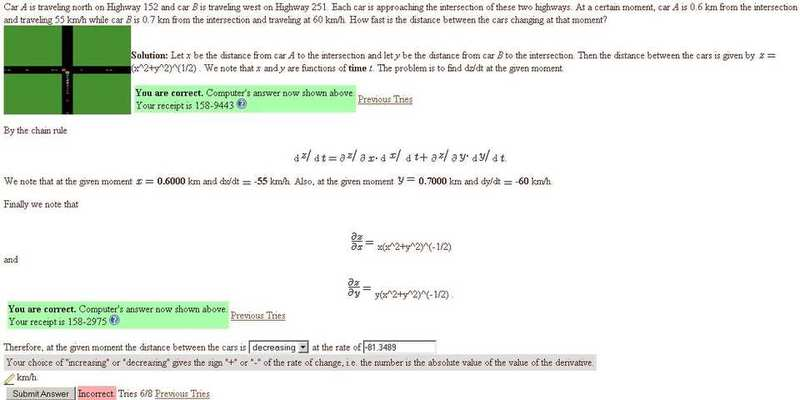# How fast is the distance between the cars changing at this moment

paraboloid
[Solved]How fast is the distance between the cars changing at this momentI'm in need of help with the last part (finding dz/dt).

I'm sorry I don't know how to use latex.
Let D refer to partial derivatives.

This was my attempt best try:

Given dz/dt = Dz/Dt*dx/d+ Dz/Dt*dy/dt and z = (x^2+y^2)^(1/2),

2z*dz/dt = 2x*dx/dt + 2y*dy/dt
2(.36+.49)^(1/2)*dz/dt= 2(.6)(-55)+2(.7)(-60)
dz/dt = (2(.6)(-55)+2(.7)(-60)) / (2(.36+.49)^(1/2))
dz/dt = -81.3489

Any help would be great,
thanks

Last edited: